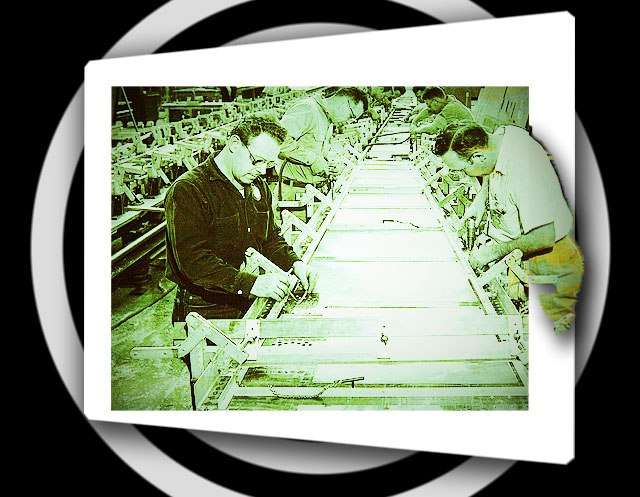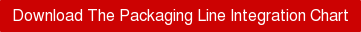By: David Roberge on September 10th, 2013

Print/Save as PDF

## Substantially reduce cost and increase production output with AutomationIf you are responsible for some or all of a manufacturing operation, you know metrics are important in determining if you are running at peak efficiency.

The introduction of automation to a packaging production line can have a substantial (positive) impact both of output and labor costs. A measure that should be part of your labor review is line realization.

## Line Realization

A percent calculation of the following:
Units per hour at which the line is set to run
_____________________________________

(Divided by)

Actual units run per hour

=Line Realization

The following is an example:

A line is set to run at 10 units per minute.

• There are 60 minutes in an hour.
• Therefore, 100% realization would be 600 units (10 x 60) per hour.
• In a given hour, 420 units are produced.
• To calculate the realization, divide the actual units produced (420) by the units that he
• line is set to run (600).
• 420 divided by 600 = .70. Therefore the realization is 70%

Our goal here is to achieve a pre-determined realization target which varies according to the line. Typically, the general aim is to strive for 70% to 75% (due to roll changes, expected downtime, etc.). If you are above 75%, consider this  far into the Green.

Improving the realization percentage has real and tangible benefits.

Some examples of these are:

• Increased profit. i.e.:
• Line labor cost/hour \$140.00
• Revenue per unit \$.70
• At 350units/hour \$245.00
• Profit for the hour \$105.00
• At 450 units per hour \$315.00>
• Profit per hour \$175.00 (revenue increase; labor remains constant)
• Hourly profit gain \$70.00
• 40 hour week \$2800.00
• 52 week year \$145,600.00
….all of which goes to offset increased operating costs. The remainder goes to EBIT.
• More timely delivery.
• Less overtime
• Faster inventory flow

## Labor Ratio

This is a percent calculation of total labor expense divided by total revenue.

Rather than focusing on a line, this focuses on the total operation. Labor expense includes all production labor as well as all support labor (supervisors, maintenance, dock workers, etc.). Anything that would reduce the number of people required or increase the efficiency of an individual would reduce the labor cost while the revenue would remain constant.

Understand how Automation can affect your line with this easy to apply chart!